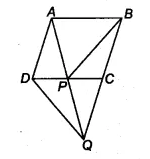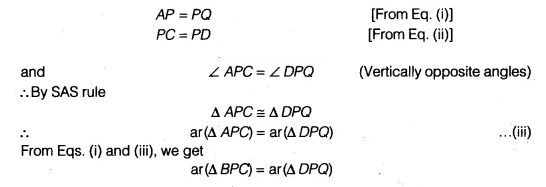# ABCD is a parallelogram and BC is produced to a point Q such that AD = CQ

In figure, ABCD is a parallelogram and BC is produced to a point Q such that AD = CQ. If AQ intersect DC at P, show that ar(BPC) = ax(DPQ).[Hint Join AC.]